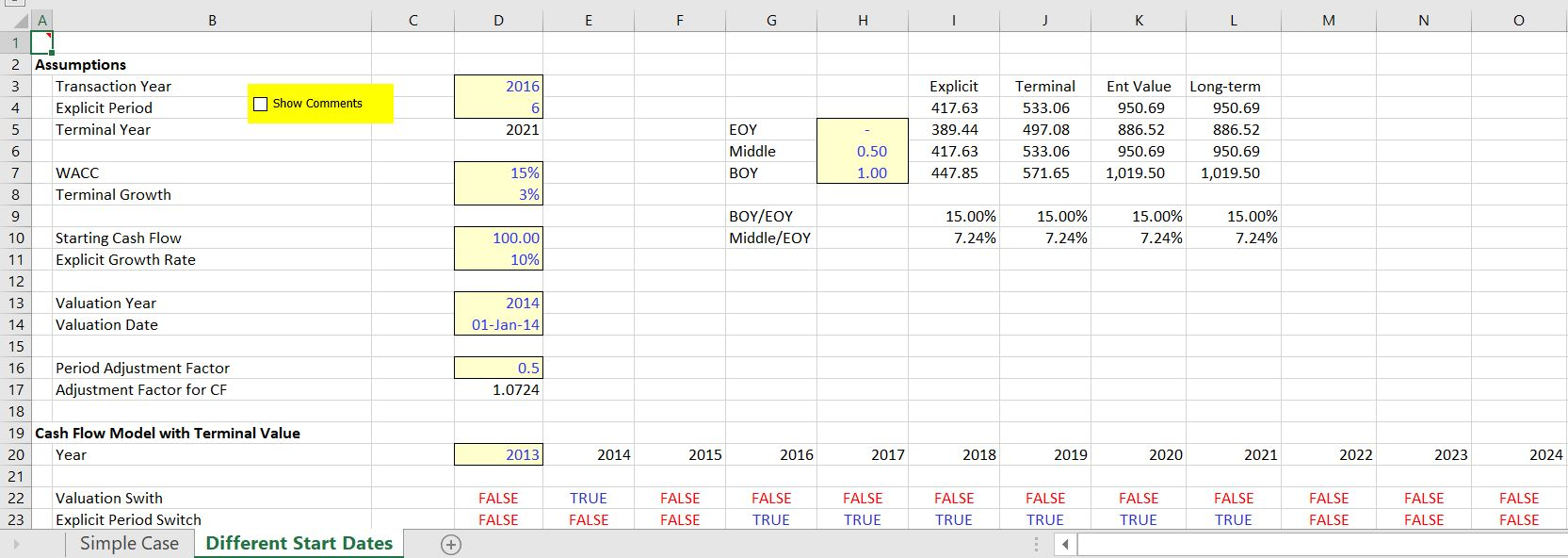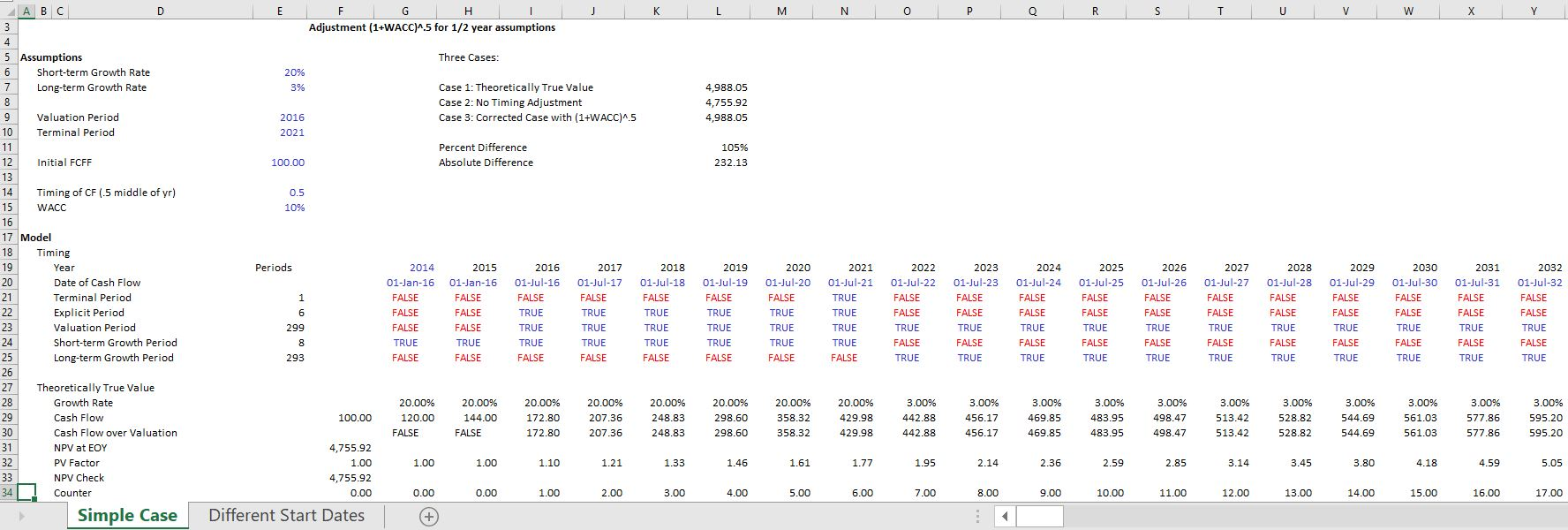# Partial Year Discounting and Timing in DCF Analysis

This page describes timing issues in a DCF analysis.  You can generally use the NPV formula that assumes end of period discounting and then multiply the result by (1+WACC)^.5 to move the result to 1/2 year convention.  I you use the NPV formula, you are implicitly assuming that all cash flow — revenues, expenses and capital occur on one day at the end of the year.  This is silly.  It would be just as silly to assume that all of the cash flows occur at the beginning of the year.  Thus a 1/2 year convention is reasonably.

The files that you can download by clicking the buttons below include proofs of this (1+WACC)^.5 formula.  As with other proofs, the analysis begins with a long-term model.  After the long-term model with the detailed partial year discounting is established, the alternative formula is established.

The first screenshot demonstrates the proof.  When both the explicit period cash flow and the terminal cash flow uses the adjustment, the explicit value plus the terminal value is the same as the theoretical long-term value.The next screenshot demonstrates how the long-term proof and the concept works.  You can set-up different TRUE and FALSE switches.  The first case is a long-term model.  Then you use the switches to make a shorter-term model with terminal value.  The value from the explicit period and the terminal period should equal the long-term value.  In the case below, the long-term value is computed with a half-year assumption.